# Python Data Types

## Introduction

In this chapter, we will discuss Python Data Types.

## Python Data Types

A data type is a classification of one of a variety of types of data, such as Number, Boolean, Strings, and so on. Data types determine the possible value for the type. If an operation is done then it can be done on the value of that type.

In the Python programming language, every value has a datatype. You don't need to declare it but you don't need to declare the datatype for that specific variable. Like in JavaScript based on the data that we assign to the variable, Python determines the type. In Python, everything is an object.

Python has many  native data types that are listed as in the following:
• Boolean: Ture or False
• Integer: Positive and Negative values like -1,-2, 0, 2, 8, and so on.
• Float: 0.2, 2.8, and so on.
• Fraction: 3/5, 2/7, and so on.
• Complex: Values that have real parts and complex parts like 2 + 3i.
• String: String is the sequence of Unicode characters
• Byte and Byte Array: This data type can save any binary files like Image(jpg, png, BMP, and so on. )
• List: an Ordered sequence of value.
• Tuples: Ordered immutable sequence of value.
• Sets: Unordered bag of values.
• Dictionaries: Unordered bags of key-value pairs.

## Boolean

Booleans are either true or false. There are two constants, True and False, that can be used to assign a value directly. Python expects an expression to evaluate to a boolean value. These places are called boolean contexts. We can write any expression in boolean contexts.

Example 1
1. a=True;
2. print(a);
Output

True

In Python, booleans can be treated as numbers. True value is 1 and a False value is 0. You can also do any operation between boolean values in Python.

Example 2
1. a=True
2. b=True
3. print(a+b)
Output

2

Because Python treats a true value as 1 and a false value as 0. Here a and b both are true so 1 + 1 = 2.

Example 3
1. a=False
2. b=True
3. print(a-b)
Output

-1

Example 4
1. a=True
2. b=False
3. print(a * b)
Output

0

Example 5
1. a=True
2. b=False
3. print(a / b)
Output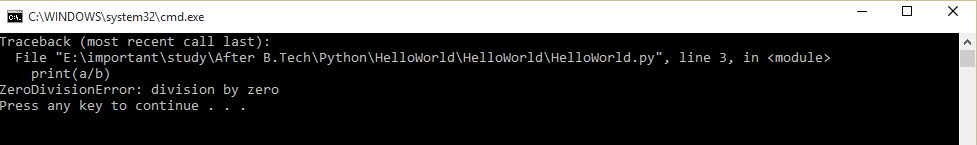## Integer

An Integer type holds an integer value or a whole number that can be a negative or positive value, like -5, -4, 0, 8, 9, 10, and so on. In the Python you can declare an integer value such as in the following:
1. a=12;
2. print(a);
Output

12

### type(variable)

But in the preceding example, you cannot determine the type of the Variable. So in Python, we have a function by which we can get the type of a variable. The function is as in the following:

Example
1. a=12
2. print(type(a))
Output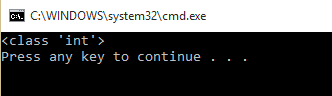We can represent any integer variable in Decimal or Octal or Hexa-Decimal format.

in Decimal

In decimal you can write directly your Python variable as in the following:
a=12

in Octal

In Octal you can represent such as in the following:

a=0O14
or
a=0o14

Example
1. a=0O14
2. print(a)
Output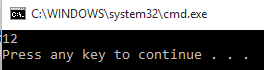In Hexa-Decimal: You can represent any integer variable as a Hexa-decimal such as in the following:

a=0XC
or
a=0xC

Example
1. a=0xC
2. print(a)
Output## Float

Float values are values that contain decimal points or a value that contains an exponent and mantissa.

Example
1. PI=3.14
2. print("Type of PI is: \n")
3. print(type(PI)) #This will print type of PI
4.
5. print("\n\nValue of PI is : %f" %PI)
Output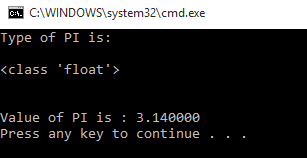We can also represent a floating-point number in E notation.

Example
1. a=1.5E+2
2. electron_charge=1.602E-19
3. speed_of_light=3.0E+8
4.
5. print(a);
6. print(electron_charge)
7. print(speed_of_light)
Output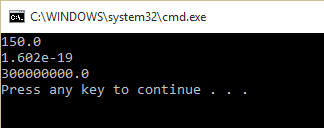## Fraction

In Python, you can also create a type that can hold fractional numbers, like 1/2, 3/5, and so on. To create a Fractional type of number you must import the "fractions" module. Then you need to create a Fraction object.

Example
1. import fractions
2. x = fractions.Fraction(13)
3. print(x)
Output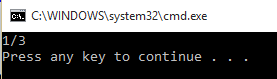Not only that, but you can also operate fractional numbers.

Example
1. import fractions
2. x = fractions.Fraction(15)
3. y = fractions.Fraction(25)
4. print("%s + %s = %s"%(x,y,(x+y)))
Output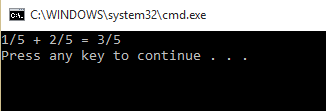"Fraction objects are automatically reduced to fractions": Assume we have a number (6/12) then it will become (1/2).

Example
1. import fractions
2. x = fractions.Fraction(612)
3. print(x)
Output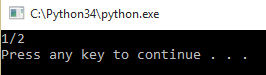"Note: You can not create a fraction with a zero denominator like 5/0 or 0/0"

## Complex Type

In Python, you can create a Complex type variable that will have a real part as well as an imaginary part, like 3 + 5i. Here the real part is 3 and the imaginary part is 5.

To create the complex type of variable in Python we have a function called complex(real_part, imaginary_part) that takes two arguments, a real part and an imaginary part.

Example
1. x = complex(3,5)
2. print(x)
Output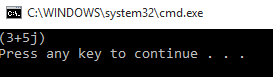You can also perform any operation on this, like:
1. x = complex(3,5)
2. y = complex(5,6)
3. print("%s + %s = %s"%(x,y,(x+y)))
Output: (3+5j) + (5+6j) = (8+11j)

## String Type

the String datatype is the same as in other languages.

Example
1. x="Hello C-Sharp Corner!"
2. print(x)
Output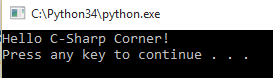## Byte and Byte Array

These objects can store binary buffers. The length of a Byte type is 1 byte, which is 8 bits. So its range will be 0 to 255. It means bytes can efficiently be one byte of data. A byte array is a type that can hold more than one byte. As we have discussed, a byte can hold the binary data so we can store any binary file in a byte array, like image, songs, and so on.

We can create any bytearray and byte variable such as in the following:
1. SomeData= 
2.
3. values = bytearray(elements)#creating Byte array
4.
5. data = bytes(elements)#creating Byte data.
In the bytearray we can modify the values as in:
1. values=4
2. values=10
But in the byte type, we cannot modify the value.
1. data=10 # This cannot be possible because Byte does not support item assignment.

## Lists Data Type

Lists are ordered sequences of values.

Creating a list:
Creating a list is very easy, you can create a Python list using square brackets and wrap comma-separated list items in square brackets.

Example
1. list=['C','C++','Java','C#']
2. print(list)
Output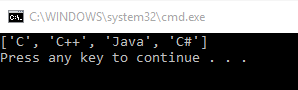You can also print the individual items of the list with its index like an array.

Example
1. list=['C','C++','Java','C#']
2. print(list)
The preceding example will print "C#".

Slicing the list: Once you have created a list you can slice that list, in other words, you can get any part of the list.

Example 1
1. list=['C','C++','Java','C#','Python']
2. print(list[1:4])
Output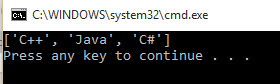Example 2
1. list=['C','C++','Java','C#','Python']
2. print(list[3:])# it will print : ['C#', 'Python']
3. print(list[:3]) # it will print : ['C', 'C++', 'Java']
Output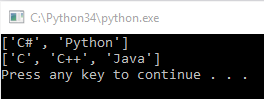Lists are mutable: we can modify the list items after we created the list.

Example
1. list=['C','C++','Java','C#','Python']
2. list='HTML'  #we can modify list item
3. print(list)
Output

HTML

## Tuples Type

A tuple is the same as a list but the difference is that a tuple is "immutable", in other words, we cannot modify a tuple after creating it.

How to create: Tuple is created the same as a list but it is enclosed in parenthesis.

Example
1. tuple=('C','C++','Java','C#','Python')
2. print(tuple)
3. tuple='HTML'# this is not possible because tuple is immutable
Output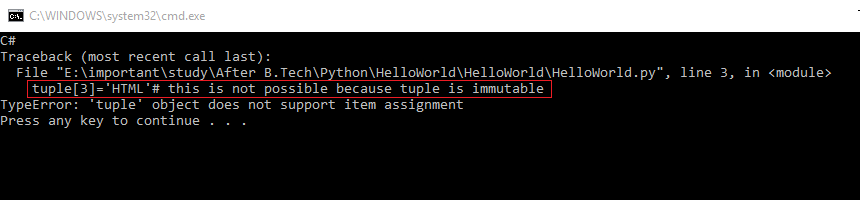## Sets

A set is a well-defined collection of distinct objects. This is also an immutable type. Once you have created a set you can perform set operations like union, intersection, and so on.

A set can be defined the same as a list but they are enclosed in curly braces ({}).

Example
1. number_set={1,2,3,4,5,6,7,8,9}
2. print(number_set)

## Dictionaries

This is an unordered set of values that has two parts, the key, and a value. In other words, a Dictionary contains key-value pairs for fast lookup of the data. We can access our data faster using a Dictionary.

Example
1. Person={'First_Name':'Sourabh','Last_Name':'Somani','Website':'http://www.sourabhsomani.com/'}
2. print(Person)
3. print(Person['First_Name'])
4. print(Person['Last_Name'])
5. print(Person['Website'])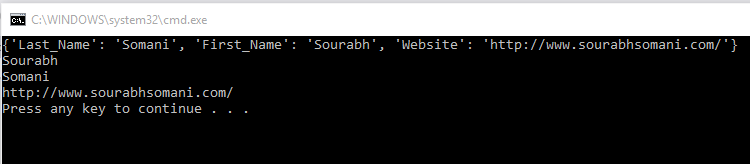## Conclusion

In the next chapter, you will learn about the print statements and escape sequences.
Next » Python Print statement and Escape Sequences